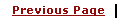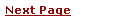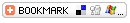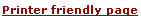C - Overview of Operator Types, Arithmetic, Bitwise, Assignment, Precedence TableLearning C
C Function References
C Useful Resources# C - Operator TypesWhat is Operator? Simple answer can be given using expression 4 + 5 is equal to 9. Here 4 and 5 are called operands and + is called operator. C language supports following type of operators.

• Arithmetic Operators

• Logical (or Relational) Operators

• Bitwise Operators

• Assignment Operators

• Misc Operators

Lets have a look on all operators one by one.

## Arithmetic Operators:

There are following arithmetic operators supported by C language:

Assume variable A holds 10 and variable B holds 20 then:

Show Examples

OperatorDescriptionExample
+Adds two operands A + B will give 30
-Subtracts second operand from the first A - B will give -10
*Multiply both operands A * B will give 200
/Divide numerator by denumerator B / A will give 2
%Modulus Operator and remainder of after an integer division B % A will give 0
++Increment operator, increases integer value by one A++ will give 11
--Decrement operator, decreases integer value by one A-- will give 9

## Logical (or Relational) Operators:

There are following logical operators supported by C language

Assume variable A holds 10 and variable B holds 20 then:

Show Examples

OperatorDescriptionExample
== Checks if the value of two operands is equal or not, if yes then condition becomes true. (A == B) is not true.
!= Checks if the value of two operands is equal or not, if values are not equal then condition becomes true. (A != B) is true.
> Checks if the value of left operand is greater than the value of right operand, if yes then condition becomes true. (A > B) is not true.
< Checks if the value of left operand is less than the value of right operand, if yes then condition becomes true. (A < B) is true.
>= Checks if the value of left operand is greater than or equal to the value of right operand, if yes then condition becomes true. (A >= B) is not true.
<= Checks if the value of left operand is less than or equal to the value of right operand, if yes then condition becomes true. (A <= B) is true.
&& Called Logical AND operator. If both the operands are non zero then then condition becomes true. (A && B) is true.
||Called Logical OR Operator. If any of the two operands is non zero then then condition becomes true. (A || B) is true.
!Called Logical NOT Operator. Use to reverses the logical state of its operand. If a condition is true then Logical NOT operator will make false. !(A && B) is false.

## Bitwise Operators:

Bitwise operator works on bits and perform bit by bit operation.

Assume if A = 60; and B = 13; Now in binary format they will be as follows:

A = 0011 1100

B = 0000 1101

-----------------

A&B = 0000 1100

A|B = 0011 1101

A^B = 0011 0001

~A  = 1100 0011

Show Examples

There are following Bitwise operators supported by C language

OperatorDescriptionExample
& Binary AND Operator copies a bit to the result if it exists in both operands. (A & B) will give 12 which is 0000 1100
| Binary OR Operator copies a bit if it exists in eather operand. (A | B) will give 61 which is 0011 1101
^ Binary XOR Operator copies the bit if it is set in one operand but not both. (A ^ B) will give 49 which is 0011 0001
~ Binary Ones Complement Operator is unary and has the efect of 'flipping' bits. (~A ) will give -60 which is 1100 0011
<< Binary Left Shift Operator. The left operands value is moved left by the number of bits specified by the right operand. A << 2 will give 240 which is 1111 0000
>> Binary Right Shift Operator. The left operands value is moved right by the number of bits specified by the right operand. A >> 2 will give 15 which is 0000 1111

## Assignment Operators:

There are following assignment operators supported by C language:

Show Examples

OperatorDescriptionExample
=Simple assignment operator, Assigns values from right side operands to left side operand C = A + B will assigne value of A + B into C
+=Add AND assignment operator, It adds right operand to the left operand and assign the result to left operand C += A is equivalent to C = C + A
-=Subtract AND assignment operator, It subtracts right operand from the left operand and assign the result to left operand C -= A is equivalent to C = C - A
*=Multiply AND assignment operator, It multiplies right operand with the left operand and assign the result to left operand C *= A is equivalent to C = C * A
/=Divide AND assignment operator, It divides left operand with the right operand and assign the result to left operand C /= A is equivalent to C = C / A
%=Modulus AND assignment operator, It takes modulus using two operands and assign the result to left operand C %= A is equivalent to C = C % A
<<=Left shift AND assignment operator C <<= 2 is same as C = C << 2
>>=Right shift AND assignment operator C >>= 2 is same as C = C >> 2
&=Bitwise AND assignment operator C &= 2 is same as C = C & 2
^=bitwise exclusive OR and assignment operator C ^= 2 is same as C = C ^ 2
|=bitwise inclusive OR and assignment operator C |= 2 is same as C = C | 2

## Short Notes on L-VALUE and R-VALUE:

x = 1; takes the value on the right (e.g. 1) and puts it in the memory referenced by x. Here x and 1 are known as L-VALUES and R-VALUES respectively L-values can be on either side of the assignment operator where as R-values only appear on the right.

So x is an L-value because it can appear on the left as we've just seen, or on the right like this: y = x; However, constants like 1 are R-values because 1 could appear on the right, but 1 = x; is invalid.

## Misc Operators

There are few other operators supported by C Language.

Show Examples

OperatorDescriptionExample
sizeof()Returns the size of an variable. sizeof(a), where a is interger, will return 4.
&Returns the address of an variable.&a; will give actaul address of the variable.
*Pointer to a variable.*a; will pointer to a variable.
? :Conditional Expression If Condition is true ? Then value X : Otherwise value Y

## Operators Categories:

All the operators we have discussed above can be categorised into following categories:

• Postfix operators, which follow a single operand.

• Unary prefix operators, which precede a single operand.

• Binary operators, which take two operands and perform a variety of arithmetic and logical operations.

• The conditional operator (a ternary operator), which takes three operands and evaluates either the second or third expression, depending on the evaluation of the first expression.

• Assignment operators, which assign a value to a variable.

• The comma operator, which guarantees left-to-right evaluation of comma-separated expressions.

## Precedence of C Operators:

Operator precedence determines the grouping of terms in an expression. This affects how an expression is evaluated. Certain operators have higher precedence than others; for example, the multiplication operator has higher precedence than the addition operator:

For example x = 7 + 3 * 2; Here x is assigned 13, not 20 because operator * has higher precedenace than + so it first get multiplied with 3*2 and then adds into 7.

Here operators with the highest precedence appear at the top of the table, those with the lowest appear at the bottom. Within an expression, higher precedenace operators will be evaluated first.

Category  Operator  Associativity
Postfix  () [] -> . ++ - -   Left to right
Unary  + - ! ~ ++ - - (type) * & sizeof  Right to left
Multiplicative   * / %  Left to right
Additive   + -  Left to right
Shift   << >>  Left to right
Relational   < <= > >=  Left to right
Equality   == !=  Left to right
Bitwise AND  Left to right
Bitwise XOR  Left to right
Bitwise OR  Left to right
Logical AND  &&  Left to right
Logical OR  ||  Left to right
Conditional  ?:  Right to left
Assignment  = += -= *= /= %= >>= <<= &= ^= |=  Right to left
Comma  Left to right